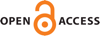Download this articleFor screen For printingRecent IssuesThe Journal About the Journal Editorial Board Editorial Interests Editorial Procedure Subscriptions Submission Guidelines Submission Page Policies for Authors Ethics Statement ISSN (electronic): 1364-0380 ISSN (print): 1465-3060 Author Index To Appear Other MSP JournalsThe infimum of the dual volume of convex cocompact hyperbolic $3$–manifolds

### Filippo Mazzoli

Geometry & Topology 27 (2023) 2319–2346##### Bibliography
 1 L Bers, Spaces of Kleinian groups, from: "Several complex variables, I" (editor J Horváth), Springer (1970) 9 MR0271333 2 F Bonahon, The geometry of Teichmüller space via geodesic currents, Invent. Math. 92 (1988) 139 MR931208 3 F Bonahon, Shearing hyperbolic surfaces, bending pleated surfaces and Thurston’s symplectic form, Ann. Fac. Sci. Toulouse Math. 5 (1996) 233 MR1413855 4 F Bonahon, A Schläfli-type formula for convex cores of hyperbolic 3–manifolds, J. Differential Geom. 50 (1998) 25 MR1678473 5 F Bonahon, Variations of the boundary geometry of 3–dimensional hyperbolic convex cores, J. Differential Geom. 50 (1998) 1 MR1678469 6 F Bonsante, J Danciger, S Maloni, J M Schlenker, The induced metric on the boundary of the convex hull of a quasicircle in hyperbolic and anti–de Sitter geometry, Geom. Topol. 25 (2021) 2827 MR4347307 7 H Brezis, Functional analysis, Sobolev spaces and partial differential equations, Springer (2011) MR2759829 8 M Bridgeman, Average bending of convex pleated planes in hyperbolic three-space, Invent. Math. 132 (1998) 381 MR1621436 9 M Bridgeman, J Brock, K Bromberg, Schwarzian derivatives, projective structures, and the Weil–Petersson gradient flow for renormalized volume, Duke Math. J. 168 (2019) 867 MR3934591 10 M Bridgeman, J Brock, K Bromberg, The Weil–Petersson gradient flow of renormalized volume and 3–dimensional convex cores, preprint (2021) arXiv:2003.00337 11 M Bridgeman, R D Canary, Bounding the bending of a hyperbolic 3–manifold, Pacific J. Math. 218 (2005) 299 MR2218349 12 J F Brock, The Weil–Petersson metric and volumes of 3–dimensional hyperbolic convex cores, J. Amer. Math. Soc. 16 (2003) 495 MR1969203 13 R D Canary, D Epstein, A Marden, editors, Fundamentals of hyperbolic geometry: selected expositions, 328, Cambridge Univ. Press (2006) MR2230672 14 L C Evans, Partial differential equations, 19, Amer. Math. Soc. (1998) MR1625845 15 A E Fischer, J E Marsden, Deformations of the scalar curvature, Duke Math. J. 42 (1975) 519 MR380907 16 F P Gardiner, N Lakic, Quasiconformal Teichmüller theory, 76, Amer. Math. Soc. (2000) MR1730906 17 M Kapovich, Hyperbolic manifolds and discrete groups, 183, Birkhäuser (2001) MR1792613 18 I Kra, On spaces of Kleinian groups, Comment. Math. Helv. 47 (1972) 53 MR306485 19 K Krasnov, J M Schlenker, On the renormalized volume of hyperbolic 3–manifolds, Comm. Math. Phys. 279 (2008) 637 MR2386723 20 K Krasnov, J M Schlenker, A symplectic map between hyperbolic and complex Teichmüller theory, Duke Math. J. 150 (2009) 331 MR2569616 21 F Labourie, Problème de Minkowski et surfaces à courbure constante dans les variétés hyperboliques, Bull. Soc. Math. France 119 (1991) 307 MR1125669 22 F Labourie, Métriques prescrites sur le bord des variétés hyperboliques de dimension 3, J. Differential Geom. 35 (1992) 609 MR1163450 23 F Labourie, Surfaces convexes dans l’espace hyperbolique et ℂP1–structures, J. London Math. Soc. 45 (1992) 549 MR1180262 24 F Labourie, Un lemme de Morse pour les surfaces convexes, Invent. Math. 141 (2000) 239 MR1775215 25 J M Lee, Introduction to Riemannian manifolds, 176, Springer (2018) MR3887684 26 A Marden, Outer circles : an introduction to hyperbolic 3–manifolds, Cambridge Univ. Press (2007) MR2355387 27 B Maskit, Self-maps on Kleinian groups, Amer. J. Math. 93 (1971) 840 MR291453 28 F Mazzoli, Constant curvature surfaces and volumes of convex co-compact hyperbolic manifolds, PhD thesis, Université du Luxembourg (2020) 29 F Mazzoli, The dual Bonahon–Schläfli formula, Algebr. Geom. Topol. 21 (2021) 279 MR4224742 30 F Mazzoli, The dual volume of quasi-Fuchsian manifolds and the Weil–Petersson distance, Trans. Amer. Math. Soc. 375 (2022) 695 MR4358680 31 F V Pallete, Continuity of the renormalized volume under geometric limits, preprint (2016) arXiv:1605.07986 32 F V Pallete, Upper bounds on renormalized volume for Schottky groups, preprint (2019) arXiv:1905.03303 33 J G Ratcliffe, Foundations of hyperbolic manifolds, 149, Springer (2006) MR2249478 34 I Rivin, J M Schlenker, On the Schlafli differential formula, preprint (2000) arXiv:math/0001176 35 H Rosenberg, J Spruck, On the existence of convex hypersurfaces of constant Gauss curvature in hyperbolic space, J. Differential Geom. 40 (1994) 379 MR1293658 36 J M Schlenker, Hypersurfaces in Hn and the space of its horospheres, Geom. Funct. Anal. 12 (2002) 395 MR1911666 37 J M Schlenker, Hyperbolic manifolds with convex boundary, Invent. Math. 163 (2006) 109 MR2208419 38 J M Schlenker, The renormalized volume and the volume of the convex core of quasifuchsian manifolds, Math. Res. Lett. 20 (2013) 773 MR3188032 39 J M Schlenker, Notes on the Schwarzian tensor and measured foliations at infinity of quasifuchsian manifolds, preprint (2017) arXiv:1708.01852 40 P A Storm, Minimal volume Alexandrov spaces, J. Differential Geom. 61 (2002) 195 MR1972145 41 P A Storm, Hyperbolic convex cores and simplicial volume, Duke Math. J. 140 (2007) 281 MR2359821 42 W P Thurston, The geometry and topology of three-manifolds, lecture notes (1979) 43 A J Tromba, Teichmüller theory in Riemannian geometry, Birkhäuser (1992) 220 MR1164870